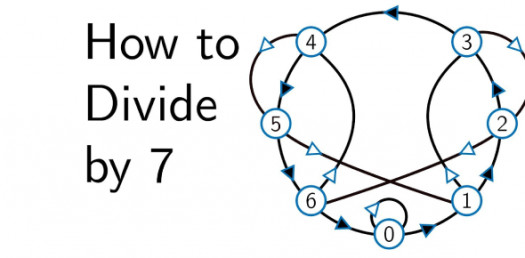Divide By 7 : Basic Division Test! Trivia Quiz

13 QuestionsSettingsThis is a 1 minute timed divided by 7 test. You must get 100% accuracy in order to move on to the next division test.

• 1.
7 ÷ 7 =
• 2.
0 ÷ 7 =
• 3.
14 ÷ 7 =
• 4.
21 ÷ 7 =
• 5.
28 ÷ 7 =
• 6.
35 ÷ 7 =
• 7.
42 ÷ 7 =
• 8.
49 ÷ 7 =
• 9.
56 ÷ 7 =
• 10.
63 ÷ 7 =
• 11.
70 ÷ 7 =
• 12.
77 ÷ 7 =
• 13.
84 ÷ 7 =
More Division Quizzes
Related Topics